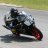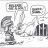# ThinkorSwim Draw Linear Regression channel with ATR width

S

#### skynetgen

##### Active member
Warehouse
I want to draw Linear Regression channel with atr width. The LR itself works fine, but ATR are curvy - i want them to be just straight lines.
What the best way to do it?

Code:
``````#channel with ATR
input price = close;
input length = 65;
input atrmod=2;
plot MiddleLR = InertiaAll(price, length);
def dist = ATR(length);
plot UpperLR = MiddleLR + 2*dist;
plot LowerLR = MiddleLR - 2*dist;``````#### RobertPayne

##### Member
I want to draw Linear Regression channel with atr width. The LR itself works fine, but ATR are curvy - i want them to be just straight lines.
What the best way to do it?
Your lines are wavy because def dist = ATR(length); is recalculated for each bar. That means that you are adding a different value to the MiddleLR at each bar.

What you want to do is add the same ATR(length) to each bar. More specifically, you want to add the ATR(length) associated with the most recent bar. Can you think of a way to do that?

Hint: you will need to use the GetValue() function.

•skynetgen#### horserider

##### Well-known member
VIP
Can also make the LRC and ATR lengths separate inputs.

input ATRLength = 14;
input NumATR = 1.5;
input fullRange = Yes;
input length = 21;#### RobertPayne

##### Member
Can also make the LRC and ATR lengths separate inputs.

input ATRLength = 14;
input NumATR = 1.5;
input fullRange = Yes;
input length = 21;
Unless I am misunderstanding how you would implement the above, I don't believe it will accomplish what @skynetgen is trying to accomplish.

When I incorporated your suggestion into his script, it still resulted in wavy lines for the same reason I described above.

Code:
``````#channel with ATR
input ATRLength = 14;
input NumATR = 1.5;
input fullRange = Yes;
input length = 21;
input price = close;
input atrmod=2;
plot MiddleLR = InertiaAll(price, length);
def dist = ATR(atrlength);
plot UpperLR = MiddleLR + 2*dist;
plot LowerLR = MiddleLR - 2*dist;``````I believe he is trying to accomplish something like this, which would be done in the manner I hinted at.@skynetgen If you can't figure it out after a little experimentation, post again and I'll tell you how I did it.

•markos#### horserider

##### Well-known member
VIP
Set ATR length higher. May not be best method but works.

Last edited:
S

#### skynetgen

##### Active member
Warehouse
Your lines are wavy because def dist = ATR(length); is recalculated for each bar. That means that you are adding a different value to the MiddleLR at each bar.

What you want to do is add the same ATR(length) to each bar. More specifically, you want to add the ATR(length) associated with the most recent bar. Can you think of a way to do that?

Hint: you will need to use the GetValue() function.
Seems this did the trick. thank you:
Code:
``````#channel with ATR
input price = HL2;
input length = 65;
input atrmod=2;
plot MiddleLR = InertiaAll(price, length);

def atrval=atr(length);
def dist = GetValue(atrval, GetMaxValueOffset(atrval, length), length);;

plot UpperLR = MiddleLR + atrmod*dist;
plot LowerLR = MiddleLR - atrmod*dist;

MiddleLR.SetDefaultColor(GetColor(5)); middleLR.setlineWeight(2);
UpperLR.SetDefaultColor(GetColor(5));
LowerLR.SetDefaultColor(GetColor(5));``````
However might not be best solution- it is a bit curly sometimes. But nowhere as bad as before though#### RobertPayne

##### Member
Seems this did the trick. thank you:
Code:
``````#channel with ATR
input price = HL2;
input length = 65;
input atrmod=2;
plot MiddleLR = InertiaAll(price, length);

def atrval=atr(length);
def dist = GetValue(atrval, GetMaxValueOffset(atrval, length), length);;

plot UpperLR = MiddleLR + atrmod*dist;
plot LowerLR = MiddleLR - atrmod*dist;

MiddleLR.SetDefaultColor(GetColor(5)); middleLR.setlineWeight(2);
UpperLR.SetDefaultColor(GetColor(5));
LowerLR.SetDefaultColor(GetColor(5));``````
However might not be best solution- it is a bit curly sometimes. But nowhere as bad as before though
If you zoom in on a chart, you will still see wavy lines when using that script.

Ruby:
``````#channel with ATR
input price = close;
input length = 65;
input atrmod=2;
def bn = barnumber();
def lastBar = highestall(if isnan(close) then 0 else bn);
def offset = bn - lastbar;
plot MiddleLR = InertiaAll(price, length);
def dist = getvalue(ATR(length),offset);
plot UpperLR = MiddleLR + 2*dist;
plot LowerLR = MiddleLR - 2*dist;``````

•markos and skynetgen#### horserider

##### Well-known member
VIP
If you zoom in on a chart, you will still see wavy lines when using that script.

Ruby:
``````#channel with ATR
input price = close;
input length = 65;
input atrmod=2;
def bn = barnumber();
def lastBar = highestall(if isnan(close) then 0 else bn);
def offset = bn - lastbar;
plot MiddleLR = InertiaAll(price, length);
def dist = getvalue(ATR(length),offset);
plot UpperLR = MiddleLR + 2*dist;
plot LowerLR = MiddleLR - 2*dist;``````

plot UpperLR = MiddleLR + atrmod*dist;
plot LowerLR = MiddleLR - atrmod*dist;

Thanks this works well for straight lines as you mentioned.

•diazlaz
S

#### skynetgen

##### Active member
Warehouse
I cannot figure out why this does not produce angle in degrees. not like trigonometry was ever my strong point but it seems straightforward yet I dont get right result:
Code:
``````def height = MiddleLR - lowest(middleLR,length);
def Angle = ATan(height/length)*180/Double.Pi;
addlabel(yes,angle +"h:"+height, if angle >anglemin then color.green else if angle<-anglemin then color.red else color.gray);``````

•RDMercer#### markos

##### Well-known member
VIP
I cannot figure out why this does not produce angle in degrees. not like trigonometry was ever my strong point but it seems straightforward yet I dont get right result:
Code:
``````def height = MiddleLR - lowest(middleLR,length);
def Angle = ATan(height/length)*180/Double.Pi;
addlabel(yes,angle +"h:"+height, if angle >anglemin then color.green else if angle<-anglemin then color.red else color.gray);``````
@skynetgen I came across something a few weeks ago on the think script lounge but I don't recall what it was exactly. However I do remember them saying that there are two types of angles. One of them is Cartesian, I don't know the name of the other one. At any rate, one of them does not work. This may seem a silly question but shouldn't anglemin have a DEF? Otherwise I don't notice a problem, maybe somebody else might.#### RobertPayne

##### Member
def height = MiddleLR - lowest(middleLR,length); def Angle = ATan(height/length)*180/Double.Pi;
Your formula is correct. However, if you are using it on an intraday chart, the numbers become ridiculously small and, practically, useless.

Compare the 1 minute chart to the daily chart below. For the 1 minute chart, the change in price (the height) is only \$0.37. When you spread that across the length of 65 bars, you get a very small angle. Whereas for the daily, you have a change in price of \$24.39 spread across the same 65 bars.All of that is to say: (1) your formula is correct and (2) it may not be useful on smaller timeframes.

•diazlaz and markos#### markos

##### Well-known member
VIP
@skynetgen Does this help?
Code:
``````# Moving Average With Slope Angle Mobius 7-25-19
# TOS Atan Example

input length = 20;
input price = close;
input averageType = AverageType.SIMPLE;

plot avg = MovingAverage(averageType, price, length);
def height = avg - avg[length];
def Angle = Atan(height/length) * 180 / Double.Pi;
addLabel(1, "Angle deg = " + Angle, color.white);``````

•diazlaz
S

#### skynetgen

##### Active member
Warehouse
Your formula is correct. However, if you are using it on an intraday chart, the numbers become ridiculously small and, practically, useless.

Compare the 1 minute chart to the daily chart below. For the 1 minute chart, the change in price (the height) is only \$0.37. When you spread that across the length of 65 bars, you get a very small angle. Whereas for the daily, you have a change in price of \$24.39 spread across the same 65 bars.All of that is to say: (1) your formula is correct and (2) it may not be useful on smaller timeframes.
Upon sleeping on it I realized this is actually much harder problem to solve: The "angle" requires some sort of normalization of period to price change. "65" length is meaningless when compared in vacuum to LR changeIndicators 0Tutorials 0